# HC Verma : Concept Of Physics Chapter 2 : Physics and Mathematics Solution PDF

HC Verma Concept Of Physics Chapter 2 (TWO) Solution is available here. You can also download it in pdf format. There are lots of consequences we have faced to publish this solution so please share it in social media.

Name of chapter 2 is Physics And Mathematics which Explains Relation between physics and mathematics. as einstein said if physics is king then definitely mathematics is queen. So Physics And Mathematics all the problem solution is covered in below given images.

### Physics And Mathematics : Chapter 2 two : Solution By HC Verma

When you read the book, you will realize that HC Varma not only tried to explains every concept in his second chapter but try to connect each concept with live problems and examples which are present in front of us so that the reader can understand concepts by himself. So download this solution of second chapter physics and mathematics from here.

• Download All Chapter Solution of HC Verma Part 1 & 2 Concept Of Physics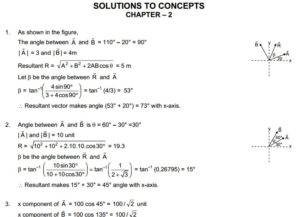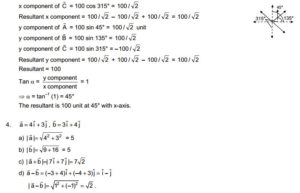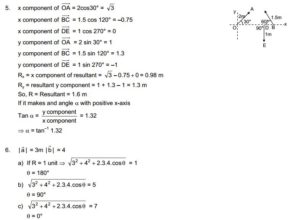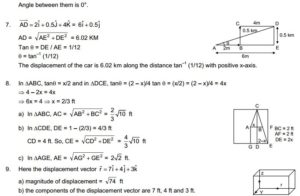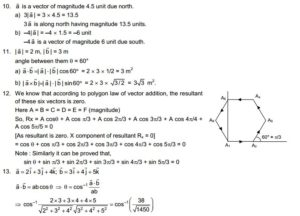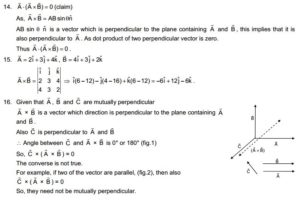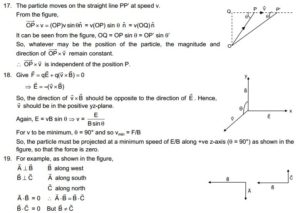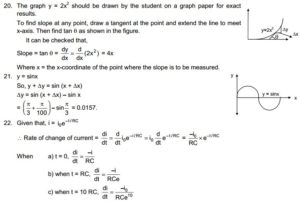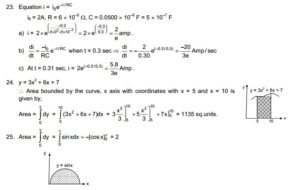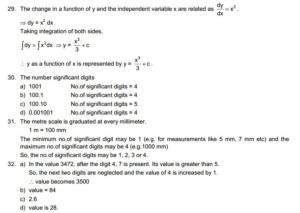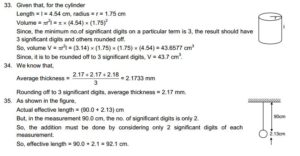Updated: November 27, 2017 — 5:17 pm

This site uses Akismet to reduce spam. Learn how your comment data is processed.